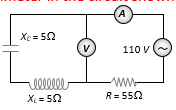Electrical Resonance series circuit
Question

# The reading of ammeter in the circuit shown will beModerate
Solution

## Given ${X}_{L}={X}_{C}=5\mathrm{\Omega }$  this is the condition of resonance. So ${\mathrm{V}}_{\mathrm{L}}={\mathrm{V}}_{\mathrm{C}},$ so net voltage across L and C combination will be zero.so current in the circuit is

Get Instant Solutions